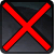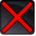# Einfacher AngriffConditions

Used by

## Related effects, buffs and debuffs

Please click on an effect below to view its details.

• [0s] [not displayed]Effect #1

 Slot: Debuff Duration: 0 Sek. Tick rate: does not tick # occurrences: 0
• On Apply

Only when the following conditions are met:

• If CASTER is positioned not more than 4m to melee distance of TARGET

Perform the following actions:

• Add effect #2 to TARGET from TARGET
• On Apply

Only when the following conditions are met:

• If CASTER is positioned 4,001m to 60m to melee distance of TARGET

Perform the following actions:

• Add effect #3 to TARGET from TARGET
• [0s] [not displayed]Effect #2

 Slot: Debuff Duration: 0 Sek. Tick rate: does not tick # occurrences: 0 Conditions: Can only by called by other effects
• On Apply

Only when the following conditions are met:

• If difficulty is set to 16-man

Perform the following actions:

• Play appearance epp . flurry . droid . atst . melee_flurry
• Weapon Damage
- Is Special Ability = (bool) false
- Ignore Dual Wield Modifier = (bool) false
- Unknown (609) = (bool) false
- Flurry Blows Min = (int) 1
- Flurry Blows Max = (int) 1
- Level Cap = (int) 0
- Threat Percent = (float) 0
- Standard Health Percent Max = (float) 0.03
- Standard Health Percent Min = (float) 0.03
- Amount Modifier Fixed Max = (float) 0
- Amount Modifier Fixed Min = (float) 0
- Amount Modifier Percent = (float) -1
- Coefficient = (float) 1
- Health Steal Percentage = (float) 0
- Slots = Int(2)
• On Apply

Only when the following conditions are met:

• If difficulty is set to 8-man HM

Perform the following actions:

• Play appearance epp . flurry . droid . atst . melee_flurry
• Weapon Damage
- Is Special Ability = (bool) false
- Ignore Dual Wield Modifier = (bool) false
- Unknown (609) = (bool) false
- Flurry Blows Min = (int) 1
- Flurry Blows Max = (int) 1
- Level Cap = (int) 0
- Threat Percent = (float) 0
- Standard Health Percent Max = (float) 0.06
- Standard Health Percent Min = (float) 0.04
- Amount Modifier Fixed Max = (float) 0
- Amount Modifier Fixed Min = (float) 0
- Amount Modifier Percent = (float) -1
- Coefficient = (float) 1
- Health Steal Percentage = (float) 0
- Slots = Int(2)
• On Apply

Only when the following conditions are met:

• If difficulty is set to 8-man SM

Perform the following actions:

• Play appearance epp . flurry . droid . atst . melee_flurry
• Weapon Damage
- Is Special Ability = (bool) false
- Ignore Dual Wield Modifier = (bool) false
- Unknown (609) = (bool) false
- Flurry Blows Min = (int) 1
- Flurry Blows Max = (int) 1
- Level Cap = (int) 0
- Threat Percent = (float) 0
- Standard Health Percent Max = (float) 0.12
- Standard Health Percent Min = (float) 0.06
- Amount Modifier Fixed Max = (float) 0
- Amount Modifier Fixed Min = (float) 0
- Amount Modifier Percent = (float) -1
- Coefficient = (float) 1
- Health Steal Percentage = (float) 0
- Slots = Int(2)
• [0s] [not displayed]Effect #3

 Slot: Debuff Duration: 0 Sek. Tick rate: does not tick # occurrences: 0 Conditions: Can only by called by other effects
• On Apply

Perform the following actions:

• Play appearance epp . flurry . droid . atst . ranged_flurry
• On Apply

Only when the following conditions are met:

• If difficulty is set to 16-man

Perform the following actions:

• Weapon Damage
- Is Special Ability = (bool) false
- Ignore Dual Wield Modifier = (bool) false
- Unknown (609) = (bool) false
- Flurry Blows Min = (int) 3
- Flurry Blows Max = (int) 3
- Level Cap = (int) 0
- Threat Percent = (float) 0
- Standard Health Percent Max = (float) 0.01
- Standard Health Percent Min = (float) 0.01
- Amount Modifier Fixed Max = (float) 0
- Amount Modifier Fixed Min = (float) 0
- Amount Modifier Percent = (float) -1
- Coefficient = (float) 1
- Health Steal Percentage = (float) 0
- Slots = Int(2)
• On Apply

Only when the following conditions are met:

• If difficulty is set to 8-man HM

Perform the following actions:

• Weapon Damage
- Is Special Ability = (bool) false
- Ignore Dual Wield Modifier = (bool) false
- Unknown (609) = (bool) false
- Flurry Blows Min = (int) 3
- Flurry Blows Max = (int) 3
- Level Cap = (int) 0
- Threat Percent = (float) 0
- Standard Health Percent Max = (float) 0.03
- Standard Health Percent Min = (float) 0.03
- Amount Modifier Fixed Max = (float) 0
- Amount Modifier Fixed Min = (float) 0
- Amount Modifier Percent = (float) -1
- Coefficient = (float) 1
- Health Steal Percentage = (float) 0
- Slots = Int(2)
• On Apply

Only when the following conditions are met:

• If difficulty is set to 8-man SM

Perform the following actions:

• Weapon Damage
- Is Special Ability = (bool) false
- Ignore Dual Wield Modifier = (bool) false
- Unknown (609) = (bool) false
- Flurry Blows Min = (int) 3
- Flurry Blows Max = (int) 3
- Level Cap = (int) 0
- Threat Percent = (float) 0
- Standard Health Percent Max = (float) 0.09
- Standard Health Percent Min = (float) 0.06
- Amount Modifier Fixed Max = (float) 0
- Amount Modifier Fixed Min = (float) 0
- Amount Modifier Percent = (float) -1
- Coefficient = (float) 1
- Health Steal Percentage = (float) 0
- Slots = Int(2)